Essential Concept 2: Standard Error of Estimate, Coefficient of Determination, Confidence Interval for a Regression Coefficient | IFT World
101 Concepts for the Level I Exam

# Essential Concept 2: Standard Error of Estimate, Coefficient of Determination, Confidence Interval for a Regression CoefficientStandard error of estimate (SEE) measures how well a given linear regression model captures the relationship between the dependent and the independent variables.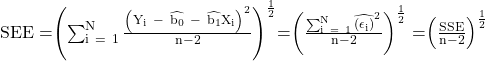The lower the SEE, the better the fit of the regression line. Also, note that the sum of the squared error terms can be written as SSE.

Coefficient of determination (R2) measures the fraction of the total variation in the dependent variable that is explained by the independent variable.

Total variation = Unexplained variation + Explained variation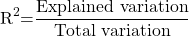In a regression with one independent variable, R2 is the square of the correlation between the dependent and independent variables. The higher the R2, the more useful the model.  has a value between 0 and 1.

The confidence interval for a regression coefficient is given by: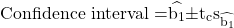where: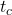is the critical t value for a given level of significance and degrees of freedom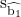is the standard error of the correlation coefficient

If the hypothesized value is outside the confidence interval, then reject the null hypothesis.